• 特点：是以起始点为中心外层层扩展（？） 基本思想： 指定起点s，即从顶点s开始计算。 引进两个集合S和U。 S：记录已求出最短路径的顶点(以及相应的最短路径长度)， U：记录还未求出最短路径的顶点(以及该...
迪杰斯特拉算法介绍
最短路径算法:用于计算一个节点到其他节点的最短路径。(一对多)特点：是以起始点为中心向外层层扩展（？）
基本思想： 指定起点s，即从顶点s开始计算。
引进两个集合S和U。
S：记录已求出最短路径的顶点(以及相应的最短路径长度)，
U：记录还未求出最短路径的顶点(以及该顶点到起点s的距离)。
-初始时，S中只有起点s；U中是除s之外的顶点。U中顶点的路径是"起点s到该顶点的路径"。然后，从U中找出路径最短的顶点v，并将其加入到S中。
-更新U中的顶点（及顶点对应的路径）。 然后，再从U中找出路径最短的顶点，并将其加入到S中。
... 重复该操作，直到遍历完所有顶点。

操作步骤
初始时，S只包含起点s；U包含除s外的其他顶点，且U中顶点的距离为"起点s到该顶点的距离"。例如，U中顶点v的距离为(s,v)的长度，然后s和v不相邻，则v的距离为∞。从U中选出"距离最短的顶点k"，并将顶点k加入到S中；同时，从U中移除顶点k。更新U中各个顶点到起点s的距离。之所以更新U中顶点的距离，是由于上一步中确定了k是求出最短路径的顶点，从而可以利用k来更新其它顶点的距离；例如，(s,v)的距离可能大于(s,k)+(k,v)的距离。重复步骤(2)和(3)，直到遍历完所有顶点 迪杰斯特拉算法图解（带权图）【下面的图是无向图】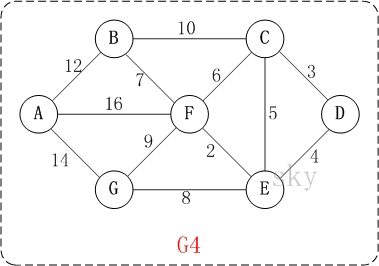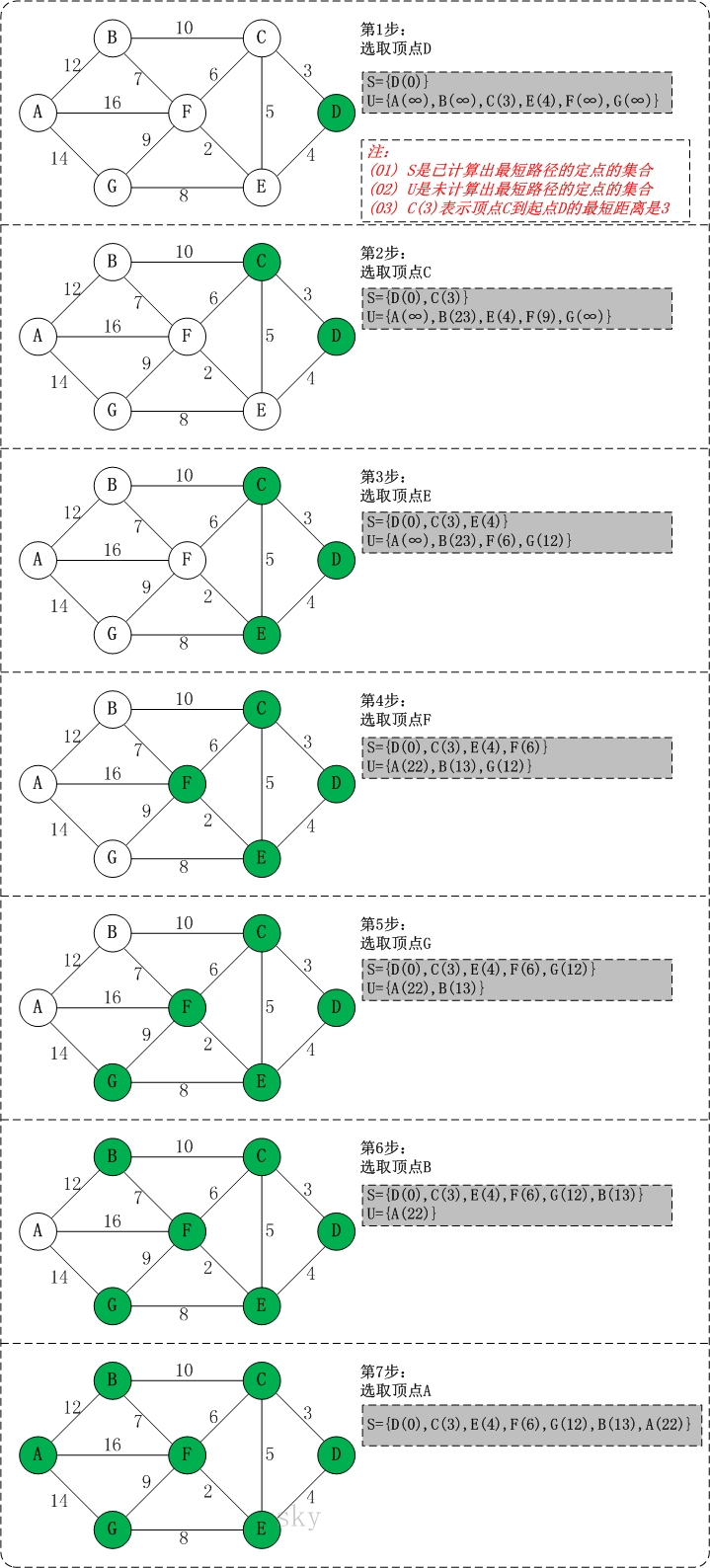初始状态：S是已计算出最短路径的顶点集合，U是未计算除最短路径的顶点的集合！
第1步：将顶点D加入到S中。 此时，S={D(0)}, U={A(∞),B(∞),C(3),E(4),F(∞),G(∞)}。 注:C(3)表示C到起点D的距离是3。
第2步：将顶点C加入到S中。 上一步操作之后，U中顶点C到起点D的距离最短；因此，将C加入到S中，同时更新U中顶点的距离。以顶点F为例，之前F到D的距离为∞；但是将C加入到S之后，F到D的距离为9=(F,C)+(C,D)。 【这里体现的是(s,v)的距离可能大于(s,k)+(k,v)的距离，从而被修改】
此时，S={D(0),C(3)}, U={A(∞),B(23),E(4),F(9),G(∞)}。

第3步：将顶点E加入到S中。 上一步操作之后，U中顶点E到起点D的距离最短；因此，将E加入到S中，同时更新U中顶点的距离。还是以顶点F为例，之前F到D的距离为9；但是将E加入到S之后，F到D的距离为6=(F,E)+(E,D)。 此时，S={D(0),C(3),E(4)}, U={A(∞),B(23),F(6),G(12)}。
第4步：将顶点F加入到S中。 此时，S={D(0),C(3),E(4),F(6)}, U={A(22),B(13),G(12)}。
第5步：将顶点G加入到S中。 此时，S={D(0),C(3),E(4),F(6),G(12)}, U={A(22),B(13)}。
第6步：将顶点B加入到S中。 此时，S={D(0),C(3),E(4),F(6),G(12),B(13)}, U={A(22)}。
第7步：将顶点A加入到S中。 此时，S={D(0),C(3),E(4),F(6),G(12),B(13),A(22)}。
此时，起点D到各个顶点的最短距离就计算出来了：

A(22) B(13) C(3) D(0) E(4) F(6) G(12)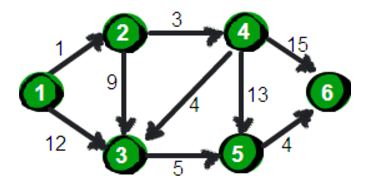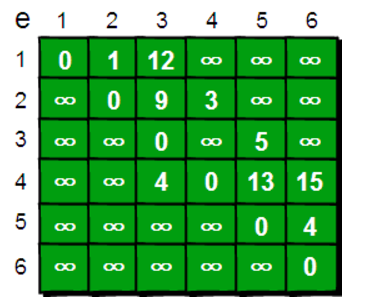const int infinity = 1000; //定义无穷常量，用1000表示

//定义图结构，采用邻接矩阵存储形式
template <int max_size>
class Graph
{
private:
/*邻接矩阵，对于有向网络（带权的有向图）其中存放的是权值*/
public:
void Dijkstra(int); //Dijkstra算法,求最短路径
};

void Graph::Dijkstra(int vertex)
{
//注意：下标表示结点
int count = 0; //用于记录访问过的结点数目,后面用于控制循环
bool find[max_size]; //用于标记已经找到最短路径的结点
int pre[max_size]; //用于存放当前结点的前驱结点的最短路径
int distance[max_size]; //用于存放当前结点的最短路径
//初始化在这里插入代码片
for(int i=0;i<max_size;i++)
pre[i] = vertex; //开始所有结点的前驱结点都是开始的vertex
for(int i=0;i<max_size;i++)
for(int i=0;i<max_size;i++)
find[i] = false; //初始化所有结点都没有找到最短路径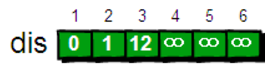find[vertex] = true;
int v = vertex; //用来迭代顶点的变量
int d; //用来表示距离
while(count < max_size)	//count用于记录访问过的次数
{
d = infinity;//开始置为无穷
for(int i=0;i<max_size;i++) //找到离最初结点最短路径的一个未访问到的结点
{
if(!find[i] && distance[i]<d) //
{
d = diatance[i];
v = i;
}
}

然后继续寻找剩下点（3,5,6）中的最小值，此处为3，也即确定了起点到点3的最近距离为distance=8,然后更新其相邻点，结果为：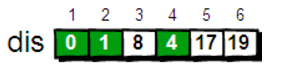find[v] = true;//表示节点放到S中

//更新剩余的结点的前驱和最短距离
for(int i=0;i<max_size;i++)
{
if(!find[i])
{
/*将上面找到的最短路径的结点作为起始点，
*连到其他未访问过的结点上，
*当比从最初结点到这个结点的路径短的时候，
*就将上个结点作为前驱结点，更新一下即可*/
if(d < distance[i])
{
pre[i] = v;
distance[i] = d;
}
}
}
count++;
}
}

总结 代码： 1.二维矩阵代表了所有节点之间的关系，但只保存权重。 2.输入是矩阵的表，输出是指定的节点到其他顶点之间的最短路径。 input:adjacent[ m] [ m] output:distance[m]
展开全文• 判断一个有向图任意两点之间是否存在路径，并输出路径BFS算法
概述
本篇博客主要内容：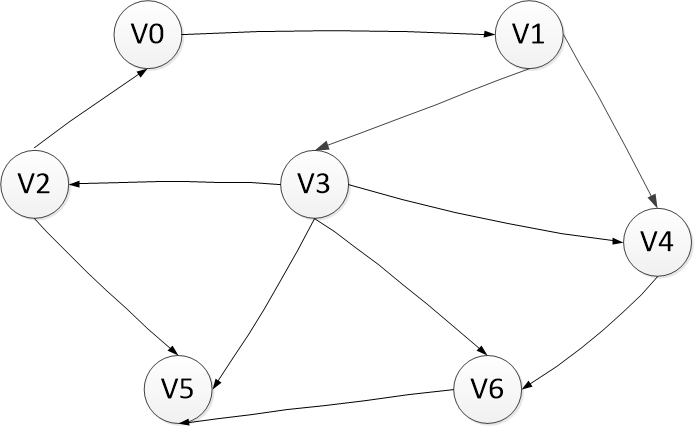选择V2作为源点，寻找距离V2距离为2的顶点，找到V2;寻找距离V2距离为1的顶点，找V2的出边，找到V0和V5；寻找距离V2距离为2的顶点，由于顶点V5的出度是0，所以找V0的出边，找到V1;寻找距离V2距离为3的顶点，找V1的出边，找到V3和V4寻找距离V2距离为4的顶点，找V3的出边，找到V5和V6于是图中所有的顶点都被访问过一次  总结一下搜索的顺序就是：V2–>V0–>V5–>V1–>V3–V4–>V5–>V6
图的邻接表实现
用邻接表实现一张图，这里采用的是两个两层链表的结构，其中一层链表存储顶点信息。每个顶点上有一个链表存储该顶点的出边。如下列伪代码所示：
#include <list>
class Edge {
// ...
};

class VertexNode {
// ...
};
class Graph {
// 顶点集合
std::list<VertexNode*> vertexSet;
//...
下面的代码给出边的定义。  边有三个属性和三个方法：  - 属性：权重、弧（有向边）的起点和终点指针；  - 方法：获取弧的起点、终点和权值
typedef class VertexNode _VertexNode;
// 边的定义
class Edge {

int weight;         /* 边的权值 */
_VertexNode * ori;  /* 弧的起点*/
_VertexNode * des;  /* 弧的终点*/

public:

Edge(int _weight,_VertexNode *_ori,_VertexNode *_des): weight(_weight),ori(_ori),des(_des){};
~Edge() {};

// 获取弧的起点
_VertexNode* getOri() {return ori;};

// 获取弧的终点
_VertexNode* getDes() {return des;};

// 获取弧的权值
int getWeight() {return weight;};

};

下面代码给出了顶点的定义。顶点具有五个属性和十个方法。属性的含义和方法的功能在代码的注释中都有说明，这里有两点说明：
VISIT_STATUS状态表示该顶点是否被访问过，解决顶点重复访问的去重；顶点定义key属性，是顶点的标号，方便给大家演示。
typedef enum  _VISIT_STATUS{
NO_VISIT,       /* 未访问过此点 */
} VISIT_STATUS;

class VertexNode {
long key;               /* 顶点的关键字，用于标识该顶点*/
int value;              /* 顶点附着的信息 */
VISIT_STATUS status;    /* 标识此顶点的访问状态 */
int distence;           /* 此顶点与搜索顶点的距离 */
std::list<Edge*>::iterator iter_edge;/* 用于遍历邻接表 */
public:

VertexNode(long _key,int _value) : key(_key), \
value(_value) { distence = 0xFFFF;status = NO_VISIT; };
~VertexNode() {};

// 获取该顶点的关键字
long getKey() { return key;};

// 设置此顶点的关键字
void setKey(long _key) { key = _key;};

// 在顶点上添加一条弧

// 在顶点上删除一条弧
void removeEdge(Edge* _edge);

// 获取邻接表第一条边
return *iter_edge;
};

bool nextEdge(Edge **_edge) {
iter_edge++;
*_edge = *iter_edge;
return true;
}
return false;
};

// 获取顶点的访问状态
VISIT_STATUS getStatus() { return status;};

// 设置顶点的访问状态
void setStatus(VISIT_STATUS _status) {status = _status;};

// 获取出发点到此点的最小距离
int getDistence() {return distence;};

// 设置出发点到此点的最小距离
void setDistence(int _distence) {distence = _distence;};

};
OKay，看过了边和顶点的定义，下面代码给出图的定义。主要有一个属性vertexSet和三个方法组成。其中vertexSet属性主要表示图中顶点的集合。
typedef std::map<long, int>  DistenceOfGraph;

class Graph {
// 顶点集合
std::list<VertexNode*> vertexSet;

public:

Graph() {};
~Graph() {};

/* 功能找出key == _searchKey的顶点到图中所有其它顶点的距离，注：不可达的顶点距离为0xFFFF
* Input <long _searchKey> 查找关键字
* Output map<long, int>&dis 输出结果，long 为关键字，int 为输出结果
*/
void bfs(long _searchKey,DistenceOfGraph &dis);

// 打印图中的全部顶点
void printNode();

// 打印顶点键值为key的边
void printEdge(long key);

// 寻找顶点关键字为key的顶点，若找到由_node变量返回
bool findNode(long key,VertexNode **_node);

// 向图中增加一个顶点

// 向图中增加一条边

};

okay，现在我们已经完成了图的邻接表存储方式的定义，以上图中的各方法将在后面具体实现。

BFS算法求解无权有向图
在本文第一部分广度优先搜索那部分给出了一个求解无权有向图的问题。下面我将结合此问题运用上面介绍的图的邻接表结构存储来介绍BFS算法的实现。
广度优先搜索过程中在访问V1顶点邻接表过程中，将V0和V5顶点后缓存，然后处理V0,再将V0顶点邻接表中的点缓存起来。这个过程用到的缓存结构其实就是一个队列。在遍历某一顶点的邻接表的同时，将邻接表中临接的顶点缓存到队列中，依次处理。  还有就是一个去除重复访问的问题。为每一个已经计算过距离的顶点，设置到达此点距离，并更新其状态由未访问过该顶点到访问过该顶点但未访问过其邻接表。  OKay，说明了这两点我们可以一起看bfs方法的代码了。
// 通过输入结点关键字_searchKey，找到该顶点
// 找到该顶点到图中其它可达顶点的最小距离
void Graph::bfs(long _searchKey,DistenceOfGraph& dis) {
queue<VertexNode*> queueCache;
int distence = 0;
// 若不存在此关键字，则返回
VertexNode *node = NULL;
if (!findNode(_searchKey, &node)) {
return ;
}

// 查找点距离查找点的距离为0
node->setDistence(0);
(dis).insert(pair<long, int>(_searchKey,0));

// 广度优先遍历图
while (IsNotNull(node)) {
// 若顶点的邻接表已经被访问，则继续访问下一个顶点
// queue next
QueueNext();
continue;
}

// 遍历顶点得全部临界顶点
while (IsNotNull(edgeTmp)) {
// 获取顶点的临接点
VertexNode * nodeTmp = edgeTmp->getDes();

// 若该顶点未访问过，则将此点加入队列，并设置到此点的距离
if (JudgeNodeStatusNoVisit(nodeTmp)) {
queueCache.push(nodeTmp);
distence = edgeTmp->getOri()->getDistence() + edgeTmp->getWeight();
(dis).insert(pair<long, int>(GetDesNodeKey((edgeTmp)),distence));
edgeTmp->getDes()->setDistence(distence);

}

EdgeNext(edgeTmp);
}

QueueNext();
}

return;
}
相信到这里，bfs算法已经很清晰了，那么我在最后给出完整的实现代码和单元测试程序。
完整代码
头文件：
#include <stdio.h>

#ifndef _GRAPH_BFS_H
#define _GRAPH_BFS_H

#include <list>
#include <map>

#define IsNotNull(a) (a)
#define IsNull(a) !(a)
#define JudgeNodeStatusNoVisit(a) (a)->getStatus() == NO_VISIT
#define GetDistence(_edge) (_edge)->getOri()->getDistence() + (_edge)->getWeight()
#define GetOriNodeKey(_edge) (_edge)->getOri()->getKey()
#define GetDesNodeKey(_edge) (_edge)->getDes()->getKey()
#define EdgeNext(_edge) if (!node->nextEdge(&(_edge))) { break;}
#define QueueNext() if (queueCache.empty()) {break;} node = queueCache.front();queueCache.pop()
#define DistenceInsert(_dis,_edge) \
DistenceOfGraph::iterator it = (_dis).find(GetOriNodeKey((_edge))); \
if (it == (_dis).end() || GetDistence((_edge)) < it->second) { \
cout<<"insert key = "<<GetOriNodeKey((_edge))<<"distence = "<<GetDistence((_edge))<<endl;\
(_dis).insert(pair<long, int>(GetOriNodeKey((_edge)),GetDistence((_edge)))); \
}

typedef class VertexNode _VertexNode;
typedef enum  _VISIT_STATUS{
NO_VISIT,       /* 未访问过此点 */
} VISIT_STATUS;
typedef std::map<long, int>DistenceOfGraph;

// 边
class Edge {

int weight;         /* 边的权值 */
_VertexNode * ori;  /* 弧的起点*/
_VertexNode * des;  /* 弧的终点*/

public:

Edge(int _weight,_VertexNode *_ori,_VertexNode *_des): weight(_weight),ori(_ori),des(_des){};
~Edge() {};

// 获取弧的起点
_VertexNode* getOri() {return ori;};

// 获取弧的终点
_VertexNode* getDes() {return des;};

// 获取弧的权值
int getWeight() {return weight;};

};

//顶点
class VertexNode {
long key;               /* 顶点的关键字，用于标识该顶点*/
int value;              /* 顶点附着的信息 */
VISIT_STATUS status;    /* 标识此顶点的访问状态 */
int distence;           /* 此顶点与搜索顶点的距离 */
std::list<Edge*>::iterator iter_edge;/* 用于遍历邻接表 */
public:

VertexNode(long _key,int _value) : key(_key), \
value(_value) { distence = 0xFFFF;status = NO_VISIT; };
~VertexNode() {};

// 获取该顶点的关键字
long getKey() { return key;};

// 设置此顶点的关键字
void setKey(long _key) { key = _key;};

// 在顶点上添加一条弧

// 在顶点上删除一条弧
void removeEdge(Edge* _edge);

// 获取邻接表第一条边
return *iter_edge;
};

bool nextEdge(Edge **_edge) {
iter_edge++;
*_edge = *iter_edge;
return true;
}
return false;
};

// 获取顶点的访问状态
VISIT_STATUS getStatus() { return status;};

// 设置顶点的访问状态
void setStatus(VISIT_STATUS _status) {status = _status;};

// 获取出发点到此点的最小距离
int getDistence() {return distence;};

// 设置出发点到此点的最小距离
void setDistence(int _distence) {distence = _distence;};

};

class Graph {
// 顶点集合
std::list<VertexNode*> vertexSet;

public:

Graph() {};
~Graph() {};

/* 功能找出key == _searchKey的顶点到图中所有其它顶点的距离，注：不可达的顶点距离为0xFFFF
* Input <long _searchKey> 查找关键字
* Output map<long, int>&dis 输出结果，long 为关键字，int 为输出结果
*/
void bfs(long _searchKey,DistenceOfGraph &dis);

// 打印图中的全部顶点
void printNode();

// 打印顶点键值为key的边
void printEdge(long key);

// 寻找顶点关键字为key的顶点，若找到由_node变量返回
bool findNode(long key,VertexNode **_node);

// 向图中增加一个顶点

// 向图中增加一条边

};

// 此测试程序测试上面Graph中的bfs方法
int testGraphBfs();
#endif

代码实现的源文件：
//
//  graph_bfs.cpp
//  100-alg-tests
//
//  Created by bobkentt on 15-8-8.
//
#include <iostream>
#include <queue>
#include <map>
#include <vector>
#include <list>
#include "graph_bfs.h"

using namespace std;

#define _DEBUG_ 1

if (IsNull(_edge)) {
exit(-1);
}

#ifdef _DEBUG_
cout<<",des's key ="<<_edge->getDes()->getKey()<<endl;;
#endif

return ;
}

bool Graph::findNode(long key,VertexNode **_node) {
list<VertexNode*> &VS = vertexSet;
list<VertexNode*>::iterator end = VS.end();
list<VertexNode*>::iterator it;
VertexNode *node = NULL;

// 遍历顶点集，找到开始结点A
for (it = VS.begin(); it != end; it++) {
node = *it;
if (node->getKey() == key)
{
break;
}
}
if (it == end) {
// 结点中
cout<<"graph::isNodeExist cannot find key = "<<key<<"in graph."<<endl;
_node = NULL;
return false;
}
*_node = node;
return true;
}

VertexNode * node = new VertexNode(key,value);

vertexSet.push_back(node);

return node;
}

void Graph::addEdge(long keyOri,long keyDes,int weight) {
VertexNode *ori = NULL;
VertexNode *des = NULL;

// 在图中查找这两个顶点
if (!findNode(keyOri, &ori) || !findNode(keyDes, &des)) {

exit(-1);
}

// 创建此弧
Edge * edge = new Edge(weight,ori,des);

// 在图中弧的起点的邻接表中，添加此弧

return ;
}

// 通过输入结点关键字_searchKey，找到该顶点
// 找到该顶点到图中其它可达顶点的最小距离
void Graph::bfs(long _searchKey,DistenceOfGraph& dis) {
queue<VertexNode*> queueCache;
int distence = 0;
// 若不存在此关键字，则返回
VertexNode *node = NULL;
if (!findNode(_searchKey, &node)) {
return ;
}

// 查找点距离查找点的距离为0
node->setDistence(0);
(dis).insert(pair<long, int>(_searchKey,0));

// 广度优先遍历图
while (IsNotNull(node)) {
// 若顶点的邻接表已经被访问，则继续访问下一个顶点
// queue next
QueueNext();
continue;
}

// 遍历顶点得全部临界顶点
while (IsNotNull(edgeTmp)) {
// 获取顶点的临接点
VertexNode * nodeTmp = edgeTmp->getDes();

// 若该顶点未访问过，则将此点加入队列，并设置到此点的距离
if (JudgeNodeStatusNoVisit(nodeTmp)) {
queueCache.push(nodeTmp);
distence = edgeTmp->getOri()->getDistence() + edgeTmp->getWeight();
(dis).insert(pair<long, int>(GetDesNodeKey((edgeTmp)),distence));
edgeTmp->getDes()->setDistence(distence);

}

EdgeNext(edgeTmp);
}

QueueNext();
}

return;
}

void Graph::printNode() {
list<VertexNode*>::iterator it = vertexSet.begin();
cout<<"The nodes of Graph's keys = ";
for (; it != vertexSet.end(); it++) {
VertexNode * node = *it;
cout<<node->getKey()<<" ";
}
cout<<endl;
return ;
}

int testGraphBfs() {
Graph G;

// 画出图中所有的点
for (int i = 0; i <= 6; i++) {
}

G.printNode();

// 画出图中所有的边

// 选择V3作为源点，求V3到其它所有的点的距离
DistenceOfGraph dis;
G.bfs(2, dis);

// debug "for each dis"
map<long,int>::iterator iter = dis.begin();
for (; iter != dis.end(); iter++) {
cout<<"key = "<<iter->first<<", dis = "<<iter->second<<endl;
}

return 0;
}

int main(int argc, const char * argv[]) {
testGraphBfs();
return 0;
}

Okay,今天就写到这里了，12点半了，困困哒，我要睡了，明天继续，这周把DFS、Dijkstra、Prim算法都实现一遍。  ps：这篇博文写的匆忙，有哪些不好，或者不对的地方请朋友们指正。
展开全文bfs 数据结构和算法 广度优先搜索
• 解决全源最短路径（对给定的G(V,E),求任意两点u，v之间的最短路径） 时间复杂度：O(n3) 2 模版 枚举顶点k ∈ [1,n] 以顶点k为中介点，枚举所有顶点对i和j（i ∈ [1,n],j ∈1[1,n]） 如果dis[i][k] + dis[k][j]...


文章目录
1 简介2 模版3 完整代码4 示例

1 简介
解决全源最短路径（对给定的图G(V,E),求任意两点u，v之间的最短路径）时间复杂度：O(n3)
2 模版
枚举顶点k ∈ [1,n]
以顶点k为中介点，枚举所有顶点对i和j（i ∈ [1,n],j ∈1[1,n]）
如果dis[i][k] + dis[k][j] <dis[i][j]成立
赋值dis[i][j] = dis[i][k] + dis[k][j]

3 完整代码
const int INF = 0x3fffffff;
const int MAXV = 200;
int N;//顶点数
int M;//边数
int dis[MAXV][MAXV];//dis[i][j]表示顶点i和顶点j的最短距离

void Floyd(){
for (int k = 0; k < N; ++k)
{
for (int i = 0; i < N; ++i)
{
for (int j = 0; j < N; ++j)
{
if(dis[i][k] != INF && dis[k][j] != INF && dis[i][k] + dis[k][j] < dis[i][j]){
dis[i][j] = dis[i][k] + dis[k][j];
}
}
}
}
}

4 示例
题目： 输入： 顶点数N 边数M 【后跟M行】 顶点i 顶点j 点i到点j的边权 输出 图中所有任意两顶点的最短距离矩阵， 矩阵中第i行第j列，表示顶点i到顶点j的最短距离 如果距离不可达，值为0x3fffffff
Sample Input:
6 8
0 1 1
0 3 4
0 4 4
1 3 2
2 5 1
3 2 2
3 4 3
4 5 3
Sample Output:
0 1 5 3 4 6
1073741823 0 4 2 5 5
1073741823 1073741823 0 1073741823 1073741823 1
1073741823 1073741823 2 0 3 3
1073741823 1073741823 1073741823 1073741823 0 3
1073741823 1073741823 1073741823 1073741823 1073741823 0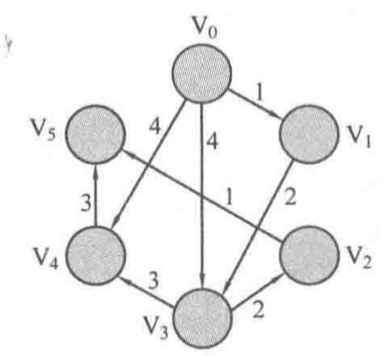完整代码
#include <cstdio>
#include <algorithm>

using std::fill;

const int INF = 0x3fffffff;
const int MAXV = 200;
int N;//顶点数
int M;//边数
int dis[MAXV][MAXV];//dis[i][j]表示顶点i和顶点j的最短距离

void Floyd(){

for (int k = 0; k < N; ++k)
{
for (int i = 0; i < N; ++i)
{
for (int j = 0; j < N; ++j)
{
if(dis[i][k] != INF && dis[k][j] != INF && dis[i][k] + dis[k][j] < dis[i][j]){
dis[i][j] = dis[i][k] + dis[k][j];
}
}
}
}
}

int main(int argc, char const *argv[])
{
fill(dis, dis + MAXV * MAXV, INF);

int u, v ,w;
scanf("%d%d", &N, &M);

for (int i = 0; i < N; ++i)
{
dis[i][i] = 0;//顶点i到顶点i的初始化距离为0
}

for (int i = 0; i < M; ++i)
{
scanf("%d%d%d", &u, &v, &w);//以有向图输入为例
dis[u][v] = w;
}

Floyd();
for (int i = 0; i < N; ++i)
{
for (int j = 0; j < N; ++j)
{
printf("%d ", dis[i][j]);
}
printf("\n");
}
return 0;
}

展开全文• 1如1所示，这是一个有向无权，如果选中某个定点作为起始顶点s，我们要找出s到其他所有顶点的最短路径问题。由于是无权的，所以我们只关心最短路径所包含的边数。这就是一个有向无权求最短路径问题，用到...

解释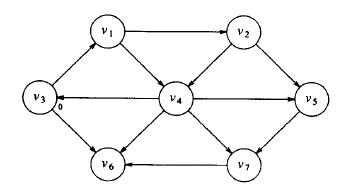图1
如图1所示，这是一个有向无权图，如果选中某个定点作为起始顶点s，我们要找出s到其他所有顶点的最短路径问题。由于是无权的，所以我们只关心最短路径所包含的边数。这就是一个有向无权图求最短路径的问题，用到BFS算法，广义优先搜索算法。
流程解析
设s为选中的v3。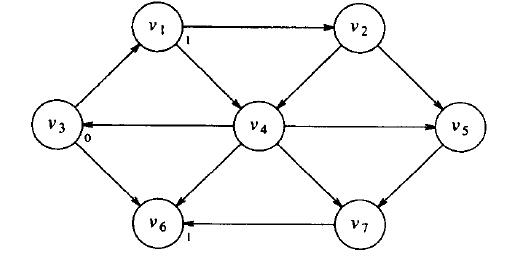从s出发路径长为1的顶点之后的图
此时可以看到从s出发到v3的最短路径长为0的路径，只有v3自己。把这个标记下来。然后寻找所有从s出发路径长为1的顶点，这些顶点可以通过考察s邻接的顶点找到。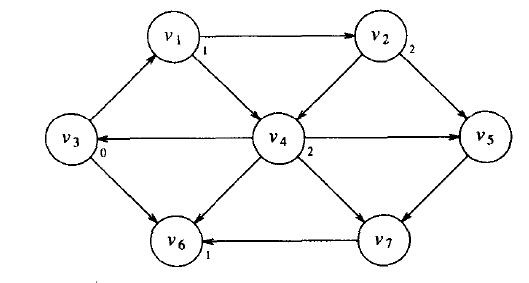找出所有从s出发路径长为1的顶点之后的图  现在寻找从s出发，最短路径为2 的顶点，找出所有邻接到v1和v6的顶点（距离为1处的顶点）。它们的最短路径还不知道，这次搜索告诉我们，到v2和v4的最短路径长为2。下图显示了到目前为止所做的工作。找出所有从s出发路径长为2的顶点之后的图
最后通过考察那些邻接到刚被赋值的v2和v4的顶点可以发现，v5和v7各有一条三边的最短路径。现在所有顶点都已经计算。下图显示最终的算法结果。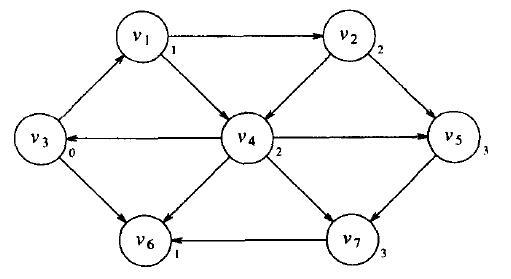下图是用于求无权最短路径计算的表的初始配置对于每个顶点，我们需要跟踪三个信息。known表示该顶点是否被处理，即求取到s的最短路径；距离d为所求取的路径长，开始被赋值无穷大，pv表示路径，通过追溯pv可以显示实际的路径长。
算法的伪代码如下
void Graph::unweighted(Vextex s)
{
for each Vertex v
{
v.dist=INFINITY;
v.known=false;
}
s.dist=0;
for(int currDist=0;currDist<NUM_VERTICES;currDist++)
for each Vertex v
if(!v.known&&v.dist==currDist)
{
v.known=true;
for each Vertex w adjacent to v
if(w.dist==INFINITY)
{
w.dist=currDist+1;
w.path=v;
}
}
}
上述代码运算复杂度是O(V^2)
下面给出使用O(V+E）复杂度的算法，类似于拓扑排序，使用连接表可以降低算法的时间复杂度为O(V+E)。
void Graph::unweighted(Vertex s)
{
Queue<Vertex> q;
for each Vertex v
v.dist=INFINITY'
s.dist=0;
q.enqueue(s);

while(!q.isEmpty())
{
Vertex v=q.dequeue();
for each Vertex w adjacent to v
if(w.dist==INFINITY)
{
w.dist=v.dist+1;
w.path=v;
q.enqueue(w);
}
}

}
上述算法的运行情况如下：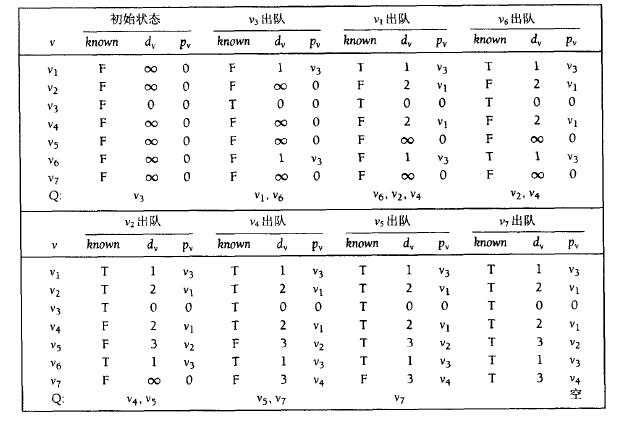实际例子
代码参考：http://blog.csdn.net/linux_ever/article/details/51305596
展开全文算法
• 所谓最短路径问题是指：如果从中某一顶点（源点）到达另一顶点（终点）的路径可能不止一条，如何找到一条路径使得沿此路径上各边的权值总和（称为路径长度）达到最小。       &...算法 java dijkstra
• 算法解决的是有向图中最短路径问题。举例来说，如果图中的顶点表示城市，而边上的权重表示著城市间开车行经的距离。 Dijkstra算法可以用来找到两个城市之间的最短路径。Dijkstra算法的输入包含了一个有权重的有向图G...算法 存储 graph path
• * 在有向图中遍历两点之间的所有路径，节点从0开始记 */ public class Main { static Scanner sc = new Scanner(System.in); static int n,m;//顶点数，边数 static int start,end;//源点，终点 static List&...
• 给定有向图，设计一个算法，找出两个结点之间是否存在一条路径 public enum State { Unvisited,Visited,Visiting; } public static boolean search(Graph g,Node start ,NLinkedList { //当作队列使用 ...
• 像这种带权的有向图，每一行都表示该行标号对应列标号的有向权值，本身到本身的数值为0，没办法到达的数值为∞ 2.最优子结构 i,j是图里面的两个不同顶点，设p为从i到j的不经过{k+1,k+2,...n}点的最短路...动态规划 贪心算法
• 问题：给定一个有向无环和一个起点，求这个点到其他点的最短路径。（参考算法导论） 因为求最短路径，所以之前的那些方法都可以用，但是这是个有向无环，可以使用更好的方法在O(V+E)的时间内求出解。在这个中...
• 如果学习x课程前必须先学习y课程，学习y课程前必须先学习z课程，学习z课程前必须先学习x课程，那么一定是有问题了...1.1检测有向环的API设计 在API中添加onStack[]布尔数组，索引为的顶点，当我们深度搜索的时： ...
• 有向图G中，如果两个顶点间至少存在一条路径，称两个顶点强连通(stronglyconnected)。如果有向图G的每两个顶点都强连通，称G是一个强连通图。非强连通图有向图的极大强连通子图，称为强连通分量(strongly ...图论算法 连通分量 Tarjan算法
• 已知一有向图G=,顶点集合V={1,2,...,n},我们可能希望确定对所有顶点对i,j ∈ V，图G中事发后都存在一条从i到 j 的路径。G的传递闭包定义为图，其中：    在Θ(n^3)时间内计算出图的传递闭包的一种方法是对E中每...算法导论 C++
• 问题描述，如所示的 解决该问题从节点1-->节点8的最短路径以及其最短距离. 利用动态规划的思想： 1、将进行分层，如所示的路径中可以分为4层，第一层为1，第二层为3/5/6，第三层为2/4/7，第四层为8 2、从...动态规划
• 本文图片及算法均来自 《算法导论》，建议阅读英文原版，里面更加详细的介绍和算法证明。 在进入Dijkstra算法之前先把书中一些关于的表达表示清楚，会更加的清晰。 G的基本表示，G = （V，E)。 V（vertex）为...算法 数据结构 dijkstra
• 给定一个有向图，判断其顶点能否到达另外一个顶点。 解决办法 使用深度优先算法，和无向图中的是一样的。 代码 import java.util.Stack; /** * Created by caipeichao on 14-6-11. */...算法 bfs dfs
• ## 最短路径问题

千次阅读 2015-10-27 15:25:53
在最短路径问题中，我们给定一个带权重的有向图G=(V, E) 和权重函数ω:E→R\omega: E \to R, 该权重函数将每条边映射到实数值的权重上，图中一条路径p=,v1,...,vk>p = , v_{1}, ... , v_{k}>的权重ω(p)\omega(p)是...数据结构
• 有向图G中,如果两个顶点间至少存在一条路径,称两个顶点强连通(strongly connected),如果有向图G的每两个顶点都强连通，称G是一个强连通图.通俗的说法是:从图G内任意一个点出发,存在通向图G内任意一点的的一条路径....强连通分量 kosaraju
• 的最短路径问题主要分为两类，单源最短路径问题和全对最短路径问题。单源最短路径问题指给点单个源点，求其到所有其它顶点之间的最短距离。而全对最短路径问题指所有顶点之间的最短路劲问题。此外对于单对最短路径...
•  深度优先搜索的基本思想是：  假设G初态为所有顶点未被访问（visited[i]=false），从G中任选一顶点vi ：  ⑴、从该顶点vi出发,首先访问vi,并置visited [vi ]=true;  ⑵、然后依次搜索vi的每一个邻接点vjC
• void Unweight( Table T) /*Assume ... /*当前无权路径长，即边数*/ Vertex V,W; /*V顶点，W顶点（两个变量）*/ for ( CurrDist = 0; CurrDist /*无权路径长的最小值是0，最大值是定点数减1*/ { for each vert数据结构 广度优先搜索
• 7.1 最短路径问题7.1.1 概述图论里最少的步骤就是最短路径问题。最短路径问题的抽象在网络中，求两个不同顶点之间的所有路径中，边的权值之和最小的那一条路径。... - （有向）有权多源最短路径问题：求任数据结构 floyd算法 dijkstra算法
• ## 无权图-最短路径

千次阅读 2017-08-22 15:55:28
如果给中的每条边赋一个值，...要求这个问题，我们首先要判断没有带负值的弧，如果是带负值，要求其最短路径比较麻烦，因为如果构成了负值圈，就可以一直循环下去，这个大小是∞的。所以本博讨论的最短路径问题
• 然后，在一幅有向图中，如果从顶点v有一条有向路径达到w，则顶点w是从顶点v可达的，但如果从w到达v的路径可能不存在。这两个顶点不是强连通的。 如果两个顶点互相可达，则它们是强连通的。如果一幅有向图中任意两...强连通分量 计算强连通分量
• 有向图G中，如果两个顶点间至少存在一条路径，称两个顶点强连通(strongly connected)。如果有向图G的每两个顶点都强连通，称G是一个强连通图。非强连通图有向图的极大强连通子图，称为强连通分量(strongly connect...
• 设G=(V,E)是一个赋权有向图，其顶点集V被划分为k个不相交的子集Vi(1)，其中，V1和Vk分别只有一个顶点s（称为源）和一个顶点t（称为汇），所有的边(u,v)的始点和终点都在相邻的两个子集Vi和Vi+1中, 且边(u动态规划
• 一，DAG算法的思想 根据结点的拓扑排序次序来对带权重的有向无环G进行边的松弛操作，在有向无环中，边可以为负值但是没有权重为负值的环，因此最短路都是存在的。二，DAG算法介绍准备阶段：一副赋值有向无环...数据结构 算法导论
• 题目7.22：试基于图的深度优先搜索策略写一算法，判别以邻接表方式存储的有向图中是否存在由顶点vi到顶点vj的路径(i≠j)。注意：算法中涉及的图的基本操作必须在此存储结构上实现。 图的邻接表结构 typedef ...数据结构...